## [Code] Image: definition, transformation, transformation=

### [Code] Image: definition, transformation, transformation=

A new Methods to Sketchup's Image Class:
image.definition
This method returns the definition of image
image.transformation
This method returns the transformation of image
image.transformation=(New Transformation)
This method applies a transformation to the image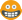Code: Select all
`class Sketchup::Image   def definition      defins = self.model.definitions      defins.each { |d| return d if d.image? && d.instances.include?(self)}; nil   end#def   def transformation      origin = self.origin      axes = self.normal.axes      tr = Geom::Transformation.axes(ORIGIN, axes.at(0), axes.at(1), axes.at(2))      tr = tr*Geom::Transformation.rotation(ORIGIN, Z_AXIS, self.zrotation)      tr = (tr*Geom::Transformation.scaling(ORIGIN, self.width/self.pixelwidth, self.height/self.pixelheight, 1)).to_a      tr = origin.x      tr = origin.y      tr = origin.z      return Geom::Transformation.new(tr)   end#def   def transformation=(tr)      self.transform! self.transformation.inverse*tr   endend#class`Use and Enjoy0

AlexMozg
PluginStore Author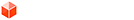### Re: [Code] Image: definition, transformation, transformation=

Awesome!
I got to try out this transformation code. I could never work it out myself. Now I might be able to hack VfSU to render Images - fooling it into thinking Images are regular components.0
Thomas Thomassen — SketchUp Monkey & Coding addictthomthom
PluginStore Author### Re: [Code] Image: definition, transformation, transformation=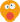whaaah! That worked sweet! VfSU now renders Images!0
Thomas Thomassen — SketchUp Monkey & Coding addictthomthom
PluginStore Author### Re: [Code] Image: definition, transformation, transformation

Thanks a lot Alex! This is very helpful.
The transformation method will return wrong result if an image is flipped (scaled in 'negative' direction).
If I will find a solution I will post it here.

Will comparison of image's normal with and normal derived from edges do the trick?
0

tomasz
SU2TH & SU2KT Developer

### Re: [Code] Image: definition, transformation, transformation

What about looking at the edge loop .reversed_in? method or something? Will that help somehow?
0
Lately you've been tan, suspicious for the winter.
All my Plugins I've writtenChris Fullmer
SketchUp Team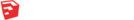### Re: [Code] Image: definition, transformation, transformation

Chris Fullmer wrote:What about looking at the edge loop .reversed_in? method or something? Will that help somehow?

I have tried it, but it doesn't work. Loop of the edges is in its 'untransformed' state stored in a image definition.

I am afraid that there is no way around it.

Btw. I didn't know, before I examined an image, that it is a 'special' ComponentDefinition (containing a face and four edges).
0

tomasz
SU2TH & SU2KT Developer

### Re: [Code] Image: definition, transformation, transformation

Is there a way to get all objects that has a vertex in a given point?

We could check whether there is the same image in a location of all face vertexes (after transformation), if not then the component would need to be flipped.
0

tomasz
SU2TH & SU2KT Developer

### Re: [Code] Image: definition, transformation, transformation

I believe you can detect a negative scaling by checking the determinant of the upper left 3x3 block matrix since all negative scalings have negative determinants unless they are simply a rotation. Here is some code.

Code: Select all
`def isflipped?(tr)    m = tr.to_a()    det = m*m*m + m*m*m + m*m*m - m*m*m - m*m*m - m*m*m    if det < 0 then        return true    end    return falseend`

Let me know if that detects the transformations you're trying to modify. However I think there is a built in ambiguity here, if you're going to interpret negative scaling as a "flip" then what direction are you flipping? I think you could rotate the image around either of the two center lines and achieve a flip but then the question is which one would you expect when you do a -1 scaling transformation? Can you clarify how the current behavior differs from the expected behavior?
0

Cleverbeans

### Re: [Code] Image: definition, transformation, transformation

Cleverbeans wrote:Let me know if that detects the transformations you're trying to modify.

The problem actually is to get the flipped transformation. The method always returns not flipped state.
It would be nice if the self.width or self.height would return negative values.
0

tomasz
SU2TH & SU2KT Developer

### Re: [Code] Image: definition, transformation, transformation

So the trouble is to get the transformation which will flip the image? Does this transformation give the behavior you're looking for? If so, do you want a transform constructed by Geom::Transformation.scaling(-1) method to produce the same behavior as this transform?

Code: Select all
`tr = Geom::Transformation.new([-1,0,0,0,0,-1,0,0,0,0,-1,0,0,0,0,1])`
0

Cleverbeans

### Re: [Code] Image: definition, transformation, transformation

The question is : Flip or not to flip and which axis? I am afraid we have no way to figure it out from Ruby alone.
Flipped.jpg

The image in the SU is flipped vertically. The transformation returned from the method will have no negative scaling along Z axis, which results in an image placed in a position marked with hidden lines.
0

tomasz
SU2TH & SU2KT Developer

### Re: [Code] Image: definition, transformation, transformation

You can get a transformation's scaling along each axis thus
[where 'tr' is the object's transformation object]
Code: Select all
`def scaleX(tr)  Math.sqrt(tr.to_a**2+tr.to_a**2+tr.to_a**2)enddef scaleY(tr)  Math.sqrt(tr.to_a**2+tr.to_a**2+tr.to_a**2)enddef scaleZ(tr)  Math.sqrt(tr.to_a**2+tr.to_a**2+tr.to_a**2)end`
This will NOT show the 'handing' BUT this should...
Code: Select all
`def flippedX?(tr)  return true if tr.to_a < 0  return falseenddef flippedY?(tr)  return true if tr.to_a < 0  return falseenddef flippedZ?(tr)  return true if tr.to_a < 0  return falseend`
I think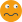- try and see - there is a way even if I've messed this up!
0
TIGTIG
Global Moderator

### Re: [Code] Image: definition, transformation, transformation

I think the methods TIG described gives the right idea, however I'm not sure there is a problem with the code as provided. It seems as though you can transform the image in any meaningful way with a correctly articulated transform, and if it is not "working" as the user expects that be an issue with the user's understanding of how transforms work rather than with the code itself.
0

Cleverbeans

### Re: [Code] Image: definition, transformation, transformation

Tomasz wrote:
Cleverbeans wrote:Let me know if that detects the transformations you're trying to modify.

The problem actually is to get the flipped transformation. The method always returns not flipped state.
It would be nice if the self.width or self.height would return negative values.

Did you find a solution that worked?
0
Thomas Thomassen — SketchUp Monkey & Coding addictthomthom
PluginStore Author### Re: [Code] Image: definition, transformation, transformation

As a 'Thanks!' to Alex and my contribution to wellness of other exporters and especially their developers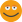here is my modified transformation method that works I think in 99.9%. It may fail due to float precision, but I looks it doesn't happen too often.

My idea is to calculate a center of the image, based on initially derived transformation and to compare it with a center of bounding box of the instance self.bounds.center. If the points are not same it means that an image is flipped.

If flipped, I create a modified transformation with a negative scaling along y axis.

Now I realized that this can be done by simply crating a Geom::Transformation.scaling origin, 1, -1, 1 and applying it to the original trans...
Code: Select all
`   def transformation      origin = self.origin      axes = self.normal.axes      tr = Geom::Transformation.axes(ORIGIN, axes.x, axes.y, axes.z)      tr = tr * Geom::Transformation.rotation(ORIGIN, Z_AXIS, self.zrotation)      tr = tr * Geom::Transformation.scaling(ORIGIN, self.width/self.pixelwidth, self.height/self.pixelheight,1)      tr=tr.to_a      tr=origin.x      tr=origin.y      tr=origin.z      trans = Geom::Transformation.new(tr) #Global! trans of an image instance      #check if flipped      center_point=Geom::Point3d.new(self.pixelwidth/2.0,self.pixelheight/2.0,0).transform! trans #center of an image      center=self.bounds.center      flipped=(center_point.x!=center.x or center_point.y!=center.y or center_point.z!=center.z)      if flipped         tr = Geom::Transformation.axes(ORIGIN, axes.x, axes.y, axes.z)         tr = tr * Geom::Transformation.rotation(ORIGIN, Z_AXIS, self.zrotation)         tr = tr * Geom::Transformation.scaling(ORIGIN, self.width/self.pixelwidth, -self.height/self.pixelheight,1)         tr=tr.to_a         tr=origin.x         tr=origin.y         tr=origin.z         trans = Geom::Transformation.new(tr)      end      return trans   end#def`
0

tomasz
SU2TH & SU2KT Developer